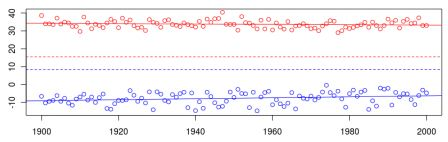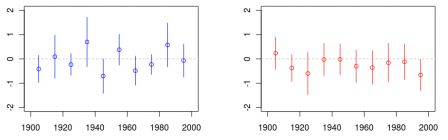Want to share your content on R-bloggers? click here if you have a blog, or here if you don't.In the paper on the heat wave in Paris (mentioned here) I discussed changes in the distribution of temperature (and autocorrelation of the time series).
During the workshop on Statistical Methods for Meteorology and Climate Change today (here) I observed that it was still an important question: is climate change affecting only averages, or does it have an impact on extremes ? And since I’ve seen nice slides to illustrate that question, I decided to play again with my dataset to see what could be said about temperature in Paris.
Recall that data can be downloaded here (daily temperature of the XXth century).
```tmaxparis=read.table("/temperature/TX_SOUID100124.txt",
Dmaxparis=as.Date(as.character(tmaxparis\$DATE),"%Y%m%d")
Tmaxparis=as.numeric(tmaxparis\$TX)/10
Dminparis=as.Date(as.character(tminparis\$DATE),"%Y%m%d")
Tminparis=as.numeric(tminparis\$TN)/10
Tminparis[Tminparis==-999.9]=NA
Tmaxparis[Tmaxparis==-999.9]=NA
annee=trunc(tminparis\$DATE/10000)
MIN=tapply(Tminparis,annee,min)
plot(unique(annee),MIN,col="blue",ylim=c(-15,40),xlim=c(1900,2000))
abline(lm(MIN~unique(annee)),col="blue")
abline(lm(Tminparis~unique(Dminparis)),col="blue",lty=2)
annee=trunc(tmaxparis\$DATE/10000)
MAX=tapply(Tmaxparis,annee,max)
points(unique(annee),MAX,col="red")
abline(lm(MAX~unique(annee)),col="red")
abline(lm(Tmaxparis~unique(Dmaxparis)),col="red",lty=2)```
On the plot below, the dots in red are the annual maximum temperatures, while the dots in blue are the annual minimum temperature. The plain line is the regression line (based on the annual max/min), and the dotted lines represent the average maximum/minimum daily temperature (to illustrate the global tendency),It is also possible to look at annual boxplot, and to focus either on minimas, or on maximas.
```annee=trunc(tminparis\$DATE/10000)
boxplot(Tminparis~as.factor(annee),ylim=c(-15,10),
xlab="Year",ylab="Temperature",col="blue")
x=boxplot(Tminparis~as.factor(annee),plot=FALSE)
xx=1:length(unique(annee))
points(xx,x\$stats[1,],pch=19,col="blue")
abline(lm(x\$stats[1,]~xx),col="blue")
annee=trunc(tmaxparis\$DATE/10000)
boxplot(Tmaxparis~as.factor(annee),ylim=c(15,40),
xlab="Year",ylab="Temperature",col="red")
x=boxplot(Tmaxparis~as.factor(annee),plot=FALSE)
xx=1:length(unique(annee))
points(xx,x\$stats[5,],pch=19,col="red")
abline(lm(x\$stats[5,]~xx),col="red")```
Plain dots are average temperature below the 5% quantile for minima, or over the 95% quantile for maxima (again with the regression line),We can observe an increasing trend on the minimas, but not on the maximas !
Finally, an alternative is to remember that we focus on annual maximas and minimas. Thus, Fisher and Tippett theory (mentioned here) can be used. Here, we fit a GEV distribution on a blog of 10 consecutive years. Recall that the GEV distribution is```install.packages("evir")
library(evir)
Pmin=Dmin=Pmax=Dmax=matrix(NA,10,3)
for(s in 1:10){
X=MIN[1:10+(s-1)*10]
FIT=gev(-X)
Pmin[s,]=FIT\$par.ests
Dmin[s,]=FIT\$par.ses
X=MAX[1:10+(s-1)*10]
FIT=gev(X)
Pmax[s,]=FIT\$par.ests
Dmax[s,]=FIT\$par.ses
}```
The location parameteris the following, with on the left the minimas and on the right the maximas,while the scale parameterisand finally the shape parameterisOn those graphs, it is very difficult to say anything regarding changes in temperature extremes… And I guess this is a reason why there is still active research on that area…